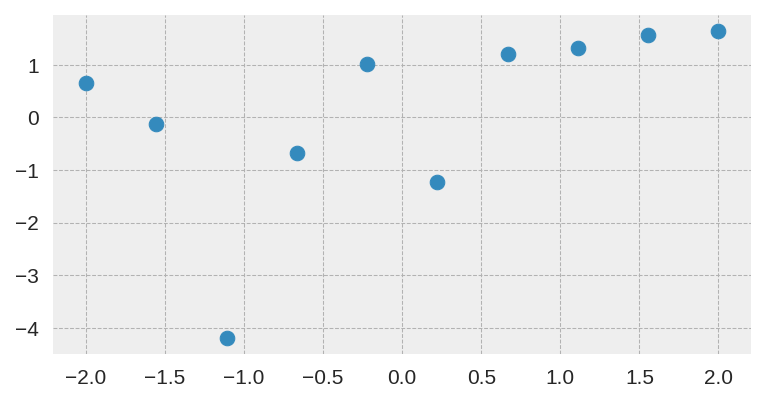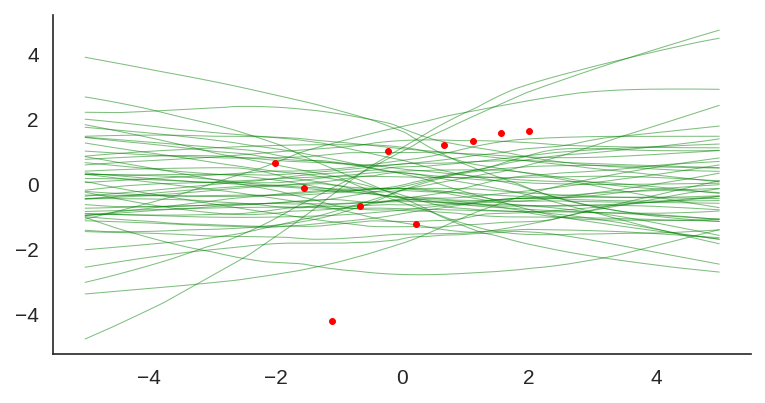## Introduction

Not a lot of people working with the Python scientific ecosystem are aware of the NEP 18 (dispatch mechanism for NumPy’s high-level array functions). Given the importance of this protocol, I decided to write this short introduction to the new dispatcher that will certainly bring a lot of benefits for the Python scientific ecosystem.

If you used PyTorch, TensorFlow, Dask, etc, you certainly noticed the similarity of their API contracts with Numpy. And it’s not by accident, Numpy’s API is one of the most fundamental and widely-used APIs for scientific computing. Numpy is so pervasive, that it ceased to be only an API and it is becoming more a protocol or an API specification.

## Benford law on GPT-2 language model

I wrote some months ago about how the Benford law emerges from language models, today I decided to evaluate the same method to check how the GPT-2 would behave with some sentences and it turns out that it seems that it is also capturing these power laws. You can find some plots with the examples below, the plots are showing the probability of the digit given a particular sentence such as “with a population size of”, showing the distribution of: $$P(\{1,2, \ldots, 9\} \vert \text{“with a population size of”})$$ for the GPT-2 medium model (345M):

## Randomized prior functions in PyTorch

I was experimenting with the approach described in “Randomized Prior Functions for Deep Reinforcement Learning” by Ian Osband et al. at NPS 2018, where they devised a very simple and practical method for uncertainty using bootstrap and randomized priors and decided to share the PyTorch code.

I really like bootstrap approaches, and in my opinion, they are usually the easiest methods to implement and provide very good posterior approximation with deep connections to Bayesian approaches, without having to deal with variational inference. They actually show in the paper that in the linear case, the method provides a Bayes posterior.

The main idea of the method is to have bootstrap to provide a non-parametric data perturbation together with randomized priors, which are nothing more than just random initialized networks.

$$Q_{\theta_k}(x) = f_{\theta_k}(x) + p_k(x)$$

The final model $$Q_{\theta_k}(x)$$ will be the k model of the ensemble that will fit the function $$f_{\theta_k}(x)$$ with an untrained prior $$p_k(x)$$.

Let’s go to the code. The first class is a simple MLP with 2 hidden layers and Glorot initialization :

class MLP(nn.Module):
def __init__(self):
super().__init__()
self.l1 = nn.Linear(1, 20)
self.l2 = nn.Linear(20, 20)
self.l3 = nn.Linear(20, 1)

nn.init.xavier_uniform_(self.l1.weight)
nn.init.xavier_uniform_(self.l2.weight)
nn.init.xavier_uniform_(self.l3.weight)

def forward(self, inputs):
x = self.l1(inputs)
x = nn.functional.selu(x)
x = self.l2(x)
x = nn.functional.selu(x)
x = self.l3(x)
return x

Then later we define a class that will take the model and the prior to produce the final model result:

class ModelWithPrior(nn.Module):
def __init__(self,
base_model : nn.Module,
prior_model : nn.Module,
prior_scale : float = 1.0):
super().__init__()
self.base_model = base_model
self.prior_model = prior_model
self.prior_scale = prior_scale

def forward(self, inputs):
prior_out = self.prior_model(inputs)
prior_out = prior_out.detach()
model_out = self.base_model(inputs)
return model_out + (self.prior_scale * prior_out)

And it’s basically that ! As you can see, it’s a very simple method, in the second part we just created a custom forward() to avoid computing/accumulating gradients for the prior network and them summing (after scaling) it with the model prediction.

To train it, you just have to use different bootstraps for each ensemble model, like in the code below:

def train_model(x_train, y_train, base_model, prior_model):
model = ModelWithPrior(base_model, prior_model, 1.0)
loss_fn = nn.MSELoss()

for epoch in range(100):
model.train()
preds = model(x_train)
loss = loss_fn(preds, y_train)

loss.backward()
optimizer.step()

return model

and using a sampler with replacement (bootstrap) as in:

dataset = TensorDataset(...)
bootstrap_sampler = RandomSampler(dataset, True, len(dataset))
batch_size=len(dataset),
sampler=bootstrap_sampler)

In this case, I used the same small dataset used in the original paper:After training it with a simple MLP prior as well, the results for the uncertainty are shown below:

If we look at just the priors, we will see the variation of the untrained networks:We can also visualize the individual model predictions showing their variability due to different initializations as well as the bootstrap noise:

Now, what is also quite interesting, is that we can change the prior to let’s say a fixed sine:

class SinPrior(nn.Module):
def forward(self, input):
return torch.sin(3 * input)

Then, when we train the same MLP model but this time using the sine prior, we can see how it affects the final prediction and uncertainty bounds:If we show each individual model, we can see the effect of the prior contribution to each individual model: